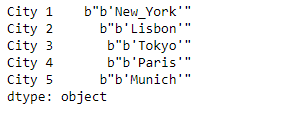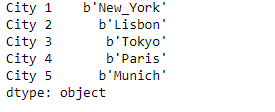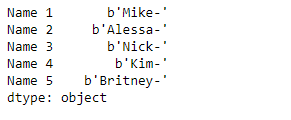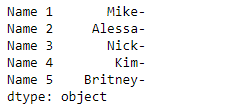# Python | Pandas Series.str.decode()

`Series.str` can be used to access the values of the series as strings and apply several methods to it. Pandas` Series.str.decode()` function is used to decode character string in the Series/Index using indicated encoding. This function is equivalent to `str.decode()` in python2 and `bytes.decode()` in python3.

Syntax: Series.str.decode(encoding, errors=’strict’)

Parameter :
encoding : str
errors : str, optional

Returns : decoded Series/Index of objects

Example #1: Use `Series.str.decode()` function to decode the character strings in the underlying data of the given series object. Use ‘UTF-8’ encoding method.

 `# importing pandas as pd ` `import` `pandas as pd ` ` `  `# Creating the Series ` `sr ``=` `pd.Series([b``"b'New_York'"``, b``"b'Lisbon'"``, b``"b'Tokyo'"``, b``"b'Paris'"``, b``"b'Munich'"``]) ` ` `  `# Creating the index ` `idx ``=` `[``'City 1'``, ``'City 2'``, ``'City 3'``, ``'City 4'``, ``'City 5'``] ` ` `  `# set the index ` `sr.index ``=` `idx ` ` `  `# Print the series ` `print``(sr) `

Output :Now we will use `Series.str.decode()` function to decode the character strings in the underlying data of the given series object.

 `# use 'UTF-8' encoding ` `result ``=` `sr.``str``.decode(encoding ``=` `'UTF-8'``) ` ` `  `# print the result ` `print``(result) `

Output :As we can see in the output, the `Series.str.decode()` function has successfully decodes the strings in the underlying data of the given series object.

Example #2 : Use `Series.str.decode()` function to decode the character strings in the underlying data of the given series object. Use ‘ASCII’ encoding method.

 `# importing pandas as pd ` `import` `pandas as pd ` ` `  `# Creating the Series ` `sr ``=` `pd.Series([b``'Mike-'``, b``'Alessa-'``, b``'Nick-'``, b``'Kim-'``, b``'Britney-'``]) ` ` `  `# Creating the index ` `idx ``=` `[``'Name 1'``, ``'Name 2'``, ``'Name 3'``, ``'Name 4'``, ``'Name 5'``] ` ` `  `# set the index ` `sr.index ``=` `idx ` ` `  `# Print the series ` `print``(sr) `

Output :Now we will use `Series.str.decode()` function to decode the character strings in the underlying data of the given series object.

 `# use 'ASCII' encoding ` `result ``=` `sr.``str``.decode(encoding ``=` `'ASCII'``) ` ` `  `# print the result ` `print``(result) `

Output :As we can see in the output, the `Series.str.decode()` function has successfully decodes the strings in the underlying data of the given series object.

Whether you're preparing for your first job interview or aiming to upskill in this ever-evolving tech landscape, GeeksforGeeks Courses are your key to success. We provide top-quality content at affordable prices, all geared towards accelerating your growth in a time-bound manner. Join the millions we've already empowered, and we're here to do the same for you. Don't miss out - check it out now!

Previous
Next